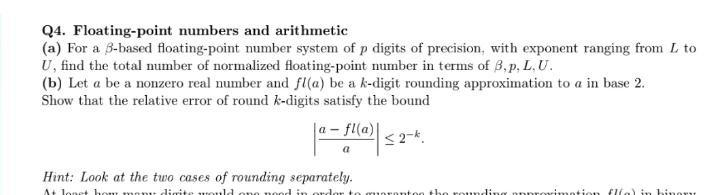Question:

# Q4. Floating-point numbers and arithmetic (a) For a B-based floating-point number system of p digits of precision, with exponentQ4. Floating-point numbers and arithmetic (a) For a B-based floating-point number system of p digits of precision, with exponent ranging from L to U, find the total mumber of normalized floating-point number in terms of B,p, L.U. (b) Let a be a nonzero real number and fl(a) be a k-digit rounding approximation to a in base 2. Show that the relative error of round k-digits satisfy the bound a-fla) 1* -f0 Ws <2-* Hint: Look at the two cases of rounding separately. A lo lo ditela the rounding arrimotid illal in hinn